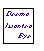P.I.G.A.L.E. Public Implementation of a Graph Algorithm Library and Editor H. de Fraysseix      P. Ossona de MendezWe develop a graph editor and a C++ algorithm library essentially concerned with planar graphs. The editor is particularly intended for graph theoretical research.
Pigale is available under the GPL license.
The source files and a Windows executable (under the non-commercial Qt license) can be obtained either by ftp or cvs at SourceForge.

It is built over a new graph data structure optimizing topological operations on static graphs.
The graphml input-output file format is partially implemented.
We also provide a Client/Server which allows, among other things, to easily interface Pigale with other programs (using a pipe).
The library includes the following new algorithms based on recent theoretical researches of our site.

• General Algorithms:
• a planarity test and an embedding computation algorithm using Fraysseix-Rosenstiehl left-right algorithm [3,5,6]
(probably the fastest planarity test ),
• a linear time algorithm to locate a Kuratowski subdivision or a cotree critical partial subgraph in a non planar graph [4,21,24],
• a linear time 3-connexity test for planar graphs [19,20],
• a linear time recognition algorithm for subdivisions of 3-connected planar graphs (),
• a linear time 4-connexity test for maximal planar graphs ,
• a fast Depth-First Search algorithm (unpublished),
• fast bipolar and regular orientation algorithms for planar graphs ,
• a linear time optimal triangulation algorithm for 3-connected planar graphs increasing the degrees by at most 6 ,
• a partitioner algorithm based on factorial analysis .
• Drawing Algorithms:
• optimized Fary drawing algorithms [8-11] relying on new planar augmentation algorithms , i.e.:
• Fraysseix, Pach Pollack algorithm,
• Schnyder algorithm using our triangulation algorithms,
• Schnyder algorithm using a vertex triangulation,
• Tutte barycentric representation of 3-connected graphs,
• A Fary representation derived from the Tutte algorithm,
• A spring embedder which preserves the map,  (unpublished).
• visibility representation of planar graphs ,
• a drawing of planar graphs using Béziers curves (based on a spring embedder, unpublished),
• an algorithm to represent 2-connected planar bipartite graphs as the incidence graph of horizontal and vertical segments [12,16],
• an algorithm to represent planar graphs by contacts of T ,
• An algorithm to represent a graph in R3, as projections of different embeddings of the graph in Rn-1 .
• Experimental Algorithms:
• an heuristic to detect symmetries ,
• an heuristic to find a maximal planar partial graph of a non planar graph (unpublished).
We are also adding some algorithms developed in other research centers:
• Gilles Schaeffer:
• random planar maps generators ,
• Nicolas Bonichon:
• random outer planar maps generators ,
• drawing of planar graphs on the grid using polylines ,
• convex drawing of 3-connected planar graphs on the grid .
• M. Matsumoto and T. Nishimura:
• Equidistributed Uniform Pseudo-Random Number Generator ,

The editor is based on the Qt library and uses the OpenGLlibrary for graph representations in R3.
Some main features of this editor are:

• constrained drawing on a grid,
• unbound number of undoes,
• macro capabilities,
• reconfigurability.Hubert de Fraysseix Patrice Ossona de Mendez